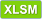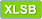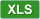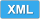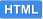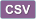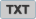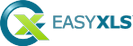# How to read XLSB file in C++.NET

 View Excel fileCopy code ``````/*------------------------------------------------------------------------------- | Tutorial 38 | | This tutorial shows how to read an Excel XLSB file in C++.NET (the | XLSB file generated by Tutorial 29 as base template), modify | some data and save it to another XLSB file (Tutorial38 - read XLSB file.xlsb). ------------------------------------------------------------------------------*/ #include "stdafx.h" using namespace System; using namespace EasyXLS; using namespace System::Data; using namespace System::IO; int main() { Console::WriteLine("Tutorial 38\n----------\n"); // Create an instance of the class that reads Excel files ExcelDocument ^workbook = gcnew ExcelDocument(); // Read XLSB file Console::WriteLine("Reading file C:\\Samples\\Tutorial29.xlsb.\n"); if (workbook->easy_LoadXLSBFile("C:\\Samples\\Tutorial29.xlsb")) { // Get the table of data for the second worksheet ExcelWorksheet ^xlsSecondTab = safe_cast(workbook->easy_getSheet("Second tab")); ExcelTable ^xlsSecondTable = xlsSecondTab->easy_getExcelTable(); // Write some data to the second sheet xlsSecondTable->easy_getCell("A1")->setValue("Data added by Tutorial38"); for (int column=0; column<5; column++) { xlsSecondTable->easy_getCell(1, column)->setValue(String::Concat("Data ", (column + 1).ToString())); } // Export the new XLSB file Console::WriteLine("Writing file C:\\Samples\\Tutorial38 - read XLSB file.xlsb."); workbook->easy_WriteXLSBFile("C:\\Samples\\Tutorial38 - read XLSB file.xlsb"); // Confirm export of Excel file String ^sError = workbook->easy_getError(); if (sError->Equals("")) Console::Write("\nFile successfully created. Press Enter to Exit..."); else Console::Write(String::Concat("\nError encountered: ", sError, "\nPress Enter to Exit...")); } else { Console::WriteLine(String::Concat("\nError reading file C:\\Samples\\Tutorial29.xlsb \n", workbook->easy_getError(), "\nPress Enter to Exit...")); } // Dispose memory delete workbook; Console::ReadLine(); return 0; } ``````

C++ without .NET Framework
This tutorial is valid for C++ applications that can use .NET Framework. For applications that does not have .NET Framework integrated, a similar code sample is available.

#### EasyXLS Excel libraries: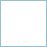##### Excel Library for C++.NET
full .NET version to import, export or convert Excel files